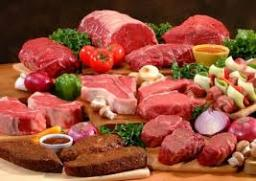# The chef

The chef has to prepare 60 hundred-gram portions of roasted pork, and he knows that 1 kg of raw meat loses 150 grams by baking. How many kilograms of meat does he have to start roasting?

x =  7.0588 kg

### Step-by-step explanation:

60·0.100= x ·(1-150/1000)

850x = 6000

x = 6000/850 = 7.05882353

x = 120/17 ≈ 7.058824

Our simple equation calculator calculates it.Did you find an error or inaccuracy? Feel free to write us. Thank you!

Tips for related online calculators
Do you have a linear equation or system of equations and looking for its solution? Or do you have a quadratic equation?
Do you want to convert mass units?